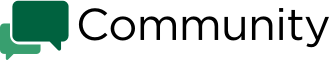# Savings account APY vs interest rate?

Savings account APY vs interest rate?
0.0 0.0 0.0 0.0 0.0 0

#1

Question: I received a reader email recently about the following scenario that could be helpful to share: A bank advertises an APY for a CD, but after getting that CD, they realize the bank uses a rounded-off interest rate. Is this normal? And, why is the actual total interest earned less than the APY would’ve calculated?

Answer: The short answer is, yes, this is normal. But the long answer can help you see how APY and interest rate work differently when calculating savings returns.

If you’re new to this topic, it might be good to start with this article on what is APY for the basic terms. But here’s a quick summary line: APY (“annual percentage yield”) is the total interest earned over one year, factoring in compound interest, while the interest rate is the interest earned before considering compound interest.

A typical compound interest calculator can show you what you can earn on a savings account or CD over time, but it’s important to enter the interest rate, not the APY, in such a calculator.

Banks advertise APY so you can compare savings accounts’ rates more easily, without needing to consider, for example, if account A has interest compounded daily while account B has interest compounded monthly (which can lead to different returns, especially if we’re talking about high balances).

But the higher the APY, the more it differs from the interest rate (thanks to more compounding happening). So for example, while 1% APY means a 0.995% interest rate, a 2% APY translates to 1.98% interest rate and a 3% APY translates to a 2.96% interest rate.

Putting the APY into a typical compound interest calculator will result in a higher return than you’d actually get, since you’re essentially factoring compounding a second time unnecessarily.

The bottom line: It’s best to check a bank’s website for an account’s interest rate, if listed beside the APY, and use that (along with the correct compounding period, usually either daily or monthly), when calculating returns over time. Happy savings!